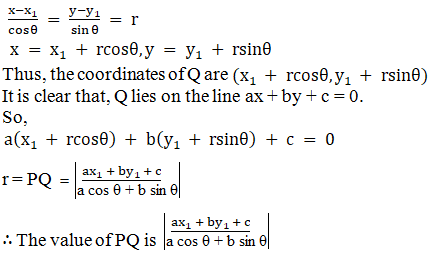# RD Sharma Solutions for Class 11 Chapter 23 - The Straight Lines Exercise 23.8

Here, in this exercise, we shall discuss one of the different forms of the equation of a straight line i.e., distance form of a line, with suitable examples for students to understand clearly. To facilitate easy learning and help students to understand the concepts explained in this chapter, RD Sharma Class 11 Maths Solutions is formulated here by our experts, as per the latest CBSE syllabus. The solutions for this exercise can be downloaded in the form of a pdf and students can use it as a reference tool to quickly review all the topics.

## Download the pdf of RD Sharma Solutions for Class 11 Maths Exercise 23.8 Chapter 23 – The Straight Lines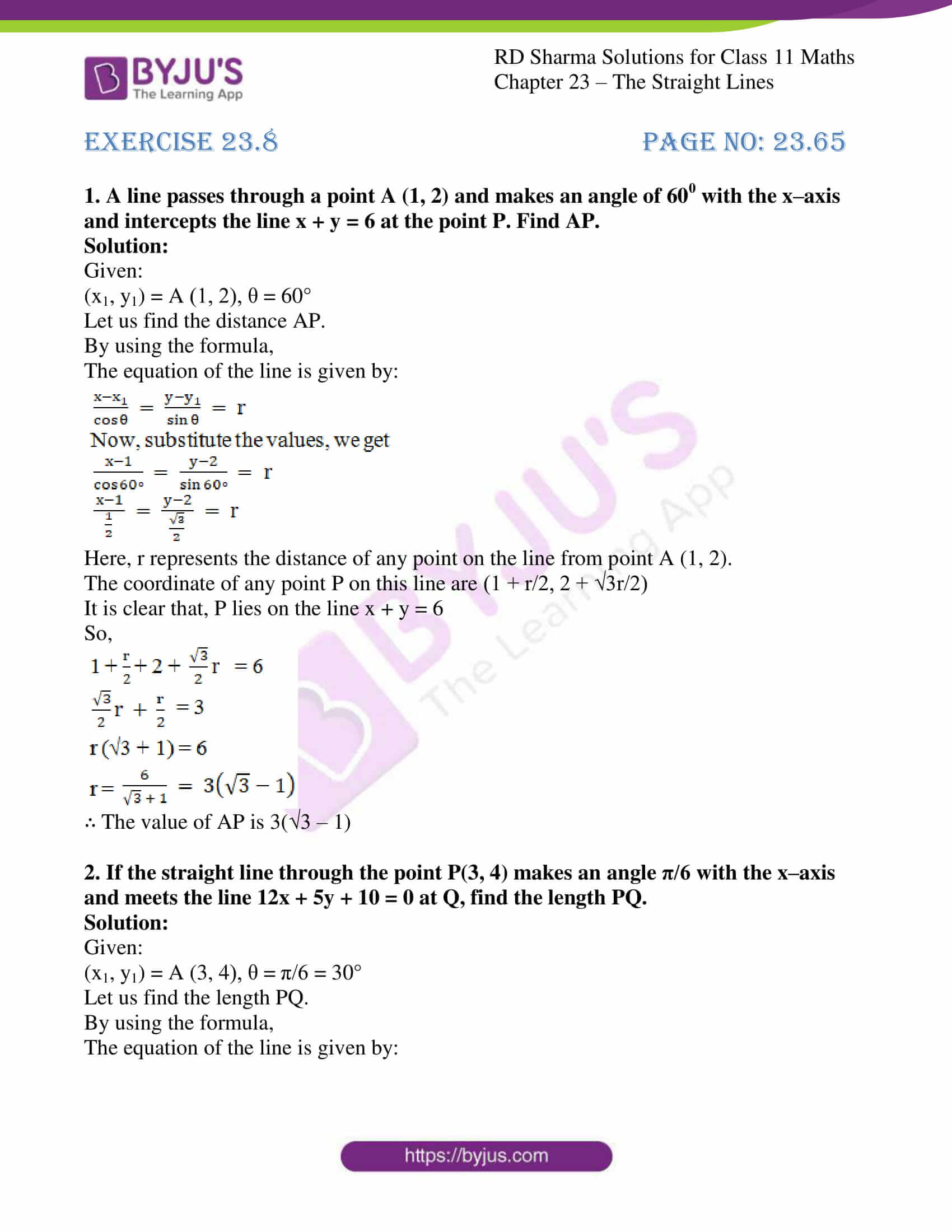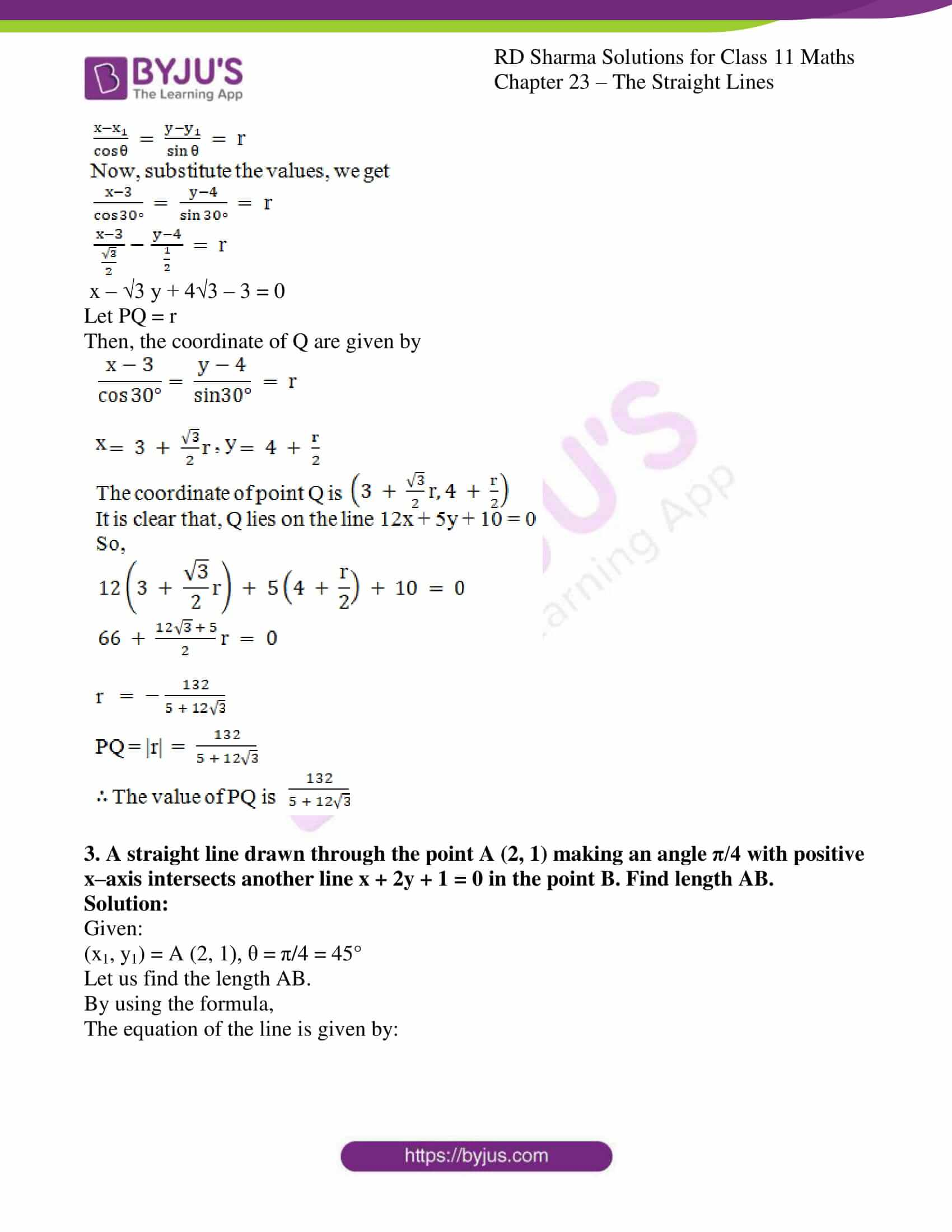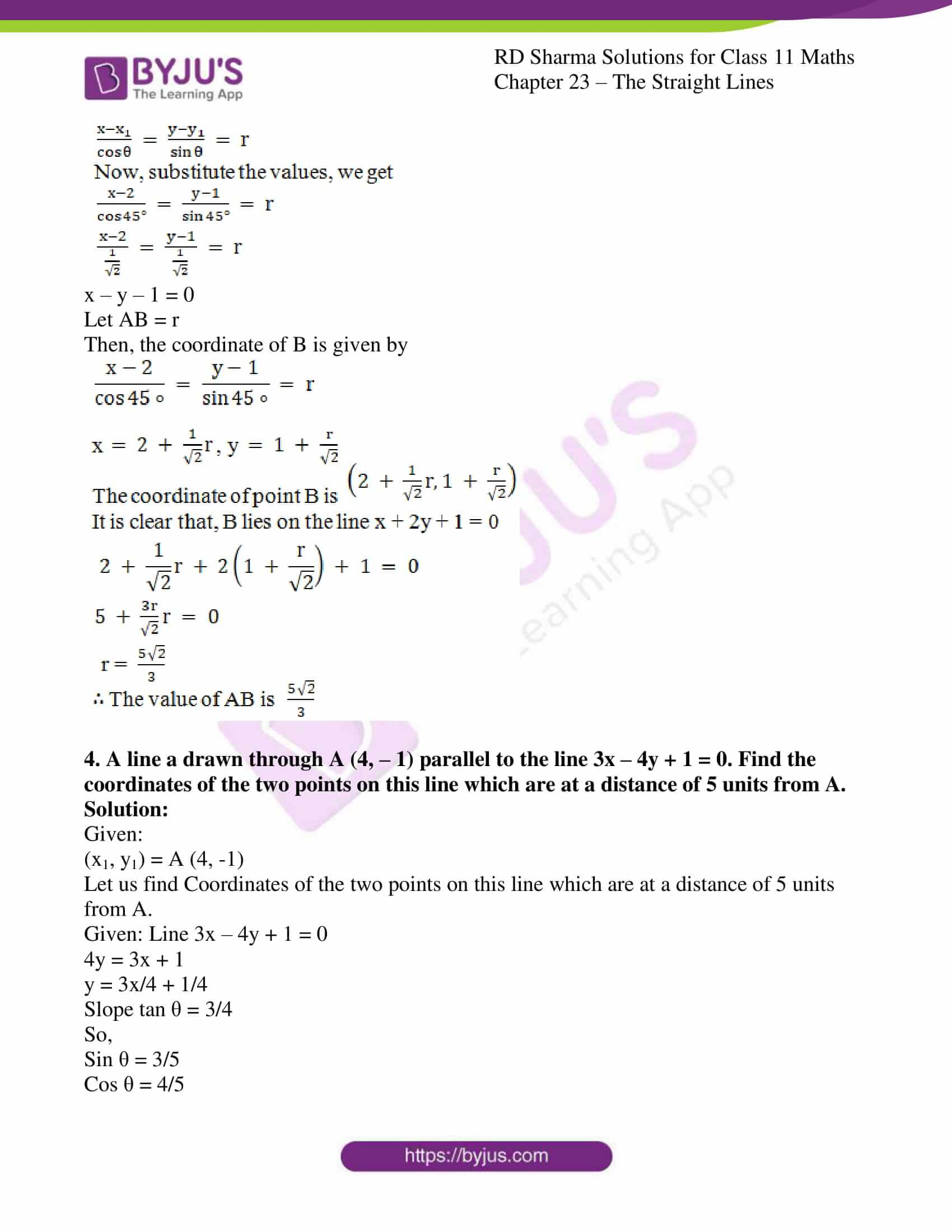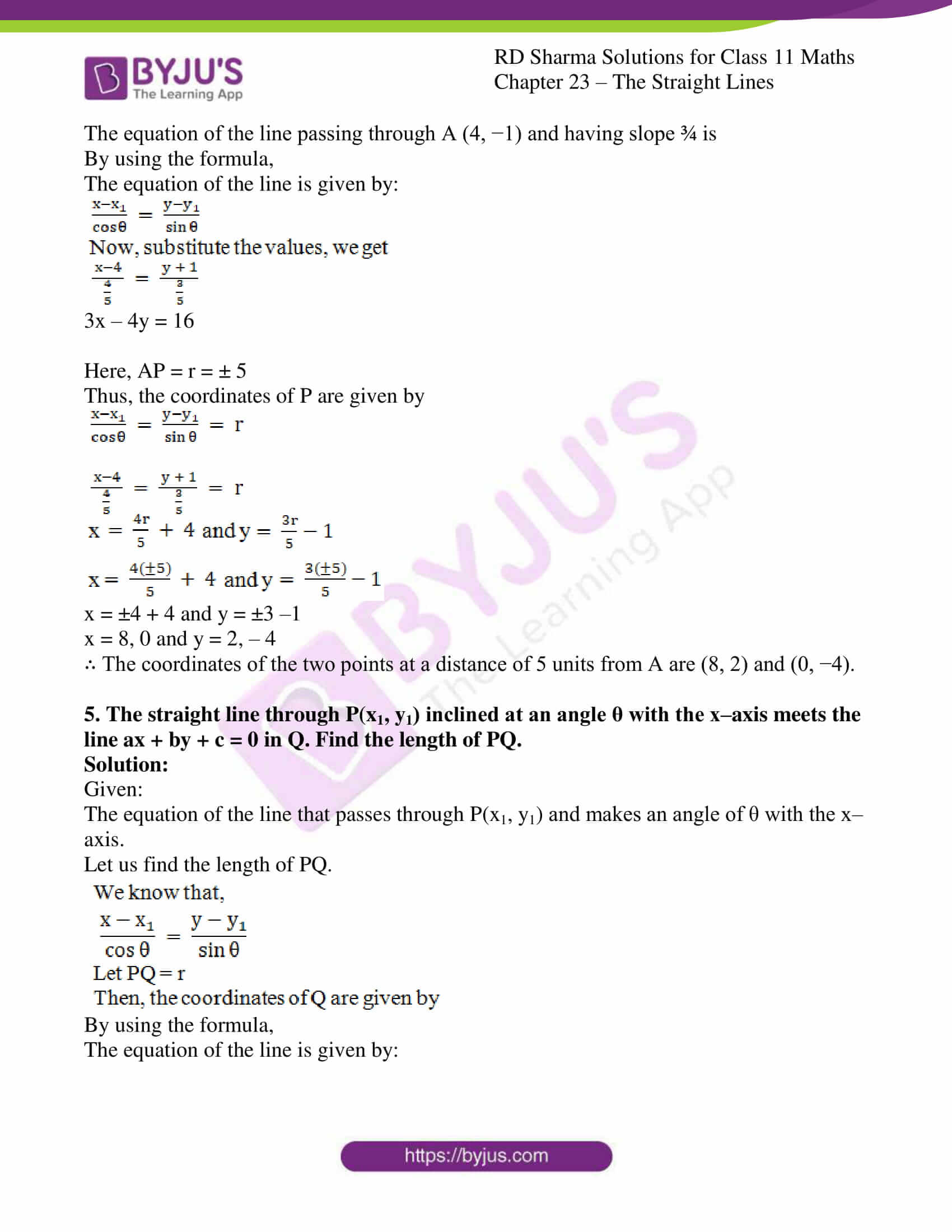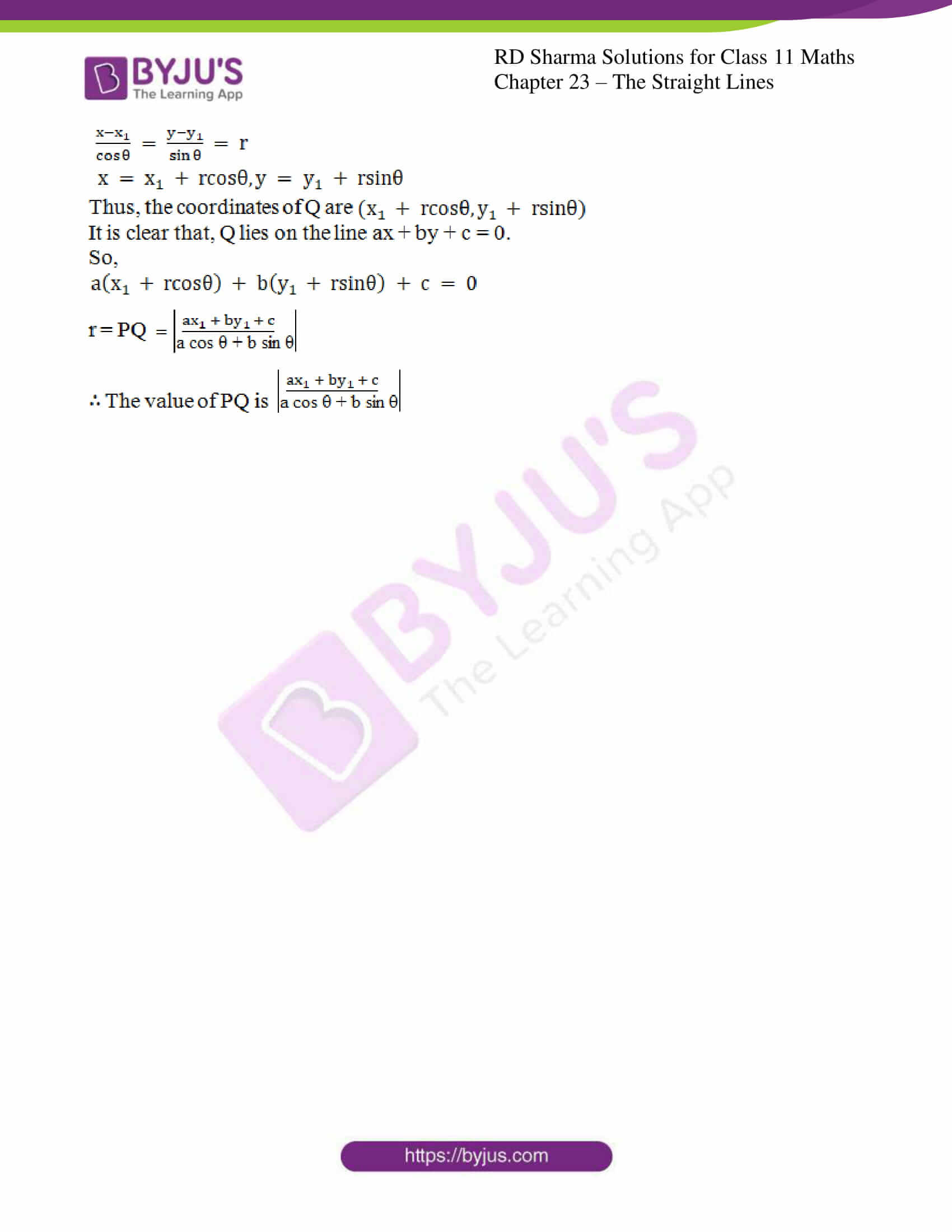### Access answers to RD Sharma Solutions for Class 11 Maths Exercise 23.8 Chapter 23 – The Straight Lines

#### EXERCISE 23.8 PAGE NO: 23.65

1. A line passes through a point A (1, 2) and makes an angle of 600 with the x–axis and intercepts the line x + y = 6 at the point P. Find AP.

Solution:

Given:

(x1, y1) = A (1, 2), θ = 60°

Let us find the distance AP.

By using the formula,

The equation of the line is given by: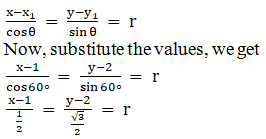Here, r represents the distance of any point on the line from point A (1, 2).

The coordinate of any point P on this line are (1 + r/2, 2 + √3r/2)

It is clear that, P lies on the line x + y = 6

So,∴ The value of AP is 3(√3 – 1)

2. If the straight line through the point P(3, 4) makes an angle π/6 with the x–axis and meets the line 12x + 5y + 10 = 0 at Q, find the length PQ.

Solution:

Given:

(x1, y1) = A (3, 4), θ = π/6 = 30°

Let us find the length PQ.

By using the formula,

The equation of the line is given by: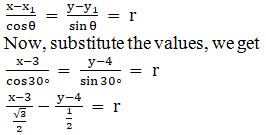x – √3 y + 4√3 – 3 = 0

Let PQ = r

Then, the coordinate of Q are given by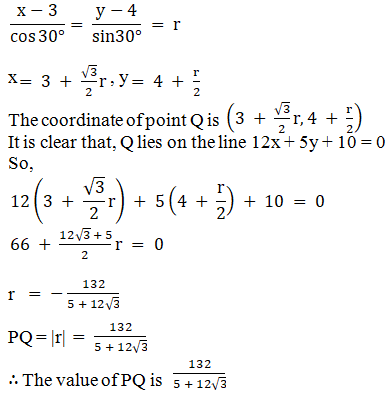3. A straight line drawn through the point A (2, 1) making an angle π/4 with positive x–axis intersects another line x + 2y + 1 = 0 in the point B. Find length AB.

Solution:

Given:

(x1, y1) = A (2, 1), θ = π/4 = 45°

Let us find the length AB.

By using the formula,

The equation of the line is given by: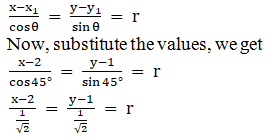x – y – 1 = 0

Let AB = r

Then, the coordinate of B is given by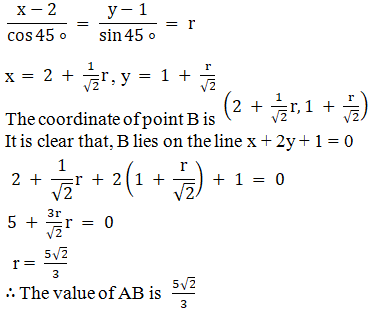4. A line a drawn through A (4, – 1) parallel to the line 3x – 4y + 1 = 0. Find the coordinates of the two points on this line which are at a distance of 5 units from A.

Solution:

Given:

(x1, y1) = A (4, -1)

Let us find Coordinates of the two points on this line which are at a distance of 5 units from A.

Given: Line 3x – 4y + 1 = 0

4y = 3x + 1

y = 3x/4 + 1/4

Slope tan θ = 3/4

So,

Sin θ = 3/5

Cos θ = 4/5

The equation of the line passing through A (4, −1) and having slope ¾ is

By using the formula,

The equation of the line is given by: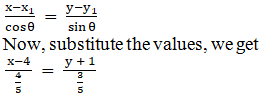3x – 4y = 16

Here, AP = r = ± 5

Thus, the coordinates of P are given by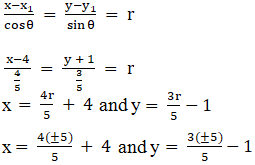x= ±4 + 4 and y = ±3 –1

x = 8, 0 and y = 2, – 4

∴ The coordinates of the two points at a distance of 5 units from A are (8, 2) and (0, −4).

5. The straight line through P(x1, y1) inclined at an angle θ with the x–axis meets the line ax + by + c = 0 in Q. Find the length of PQ.

Solution:

Given:

The equation of the line that passes through P(x1, y1) and makes an angle of θ with the x–axis.

Let us find the length of PQ.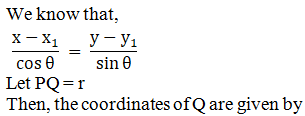By using the formula,

The equation of the line is given by: